JEE  >  Test: Single Correct MCQs: Work, Energy and Power | JEE Advanced

# Test: Single Correct MCQs: Work, Energy and Power | JEE Advanced

Test Description

## 21 Questions MCQ Test Physics For JEE | Test: Single Correct MCQs: Work, Energy and Power | JEE Advanced

Test: Single Correct MCQs: Work, Energy and Power | JEE Advanced for JEE 2023 is part of Physics For JEE preparation. The Test: Single Correct MCQs: Work, Energy and Power | JEE Advanced questions and answers have been prepared according to the JEE exam syllabus.The Test: Single Correct MCQs: Work, Energy and Power | JEE Advanced MCQs are made for JEE 2023 Exam. Find important definitions, questions, notes, meanings, examples, exercises, MCQs and online tests for Test: Single Correct MCQs: Work, Energy and Power | JEE Advanced below.
Solutions of Test: Single Correct MCQs: Work, Energy and Power | JEE Advanced questions in English are available as part of our Physics For JEE for JEE & Test: Single Correct MCQs: Work, Energy and Power | JEE Advanced solutions in Hindi for Physics For JEE course. Download more important topics, notes, lectures and mock test series for JEE Exam by signing up for free. Attempt Test: Single Correct MCQs: Work, Energy and Power | JEE Advanced | 21 questions in 30 minutes | Mock test for JEE preparation | Free important questions MCQ to study Physics For JEE for JEE Exam | Download free PDF with solutions
 1 Crore+ students have signed up on EduRev. Have you?
Test: Single Correct MCQs: Work, Energy and Power | JEE Advanced - Question 1

### If a machine is lubricated with oil (1980)

Detailed Solution for Test: Single Correct MCQs: Work, Energy and Power | JEE Advanced - Question 1

Mechanical efficiency =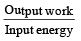The output work will increase because the friction becomes less. Thus the mechanical efficiency increases.

Test: Single Correct MCQs: Work, Energy and Power | JEE Advanced - Question 2

### Two masses of 1 gm and 4 gm are moving with equal kinetic energies. The ratio of the magnitudes of their linear momenta is

Detailed Solution for Test: Single Correct MCQs: Work, Energy and Power | JEE Advanced - Question 2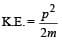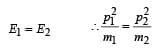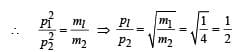Test: Single Correct MCQs: Work, Energy and Power | JEE Advanced - Question 3

### A particle of mass m is moving in a circular path of constant radius r such that its centripetal acceleration ac is  varying with time t as ac = k2rt2 where k is  a constant. The  power delivered to the particles by the force acting on it is:

Detailed Solution for Test: Single Correct MCQs: Work, Energy and Power | JEE Advanced - Question 3

The centripetal acceleration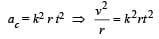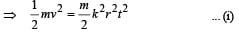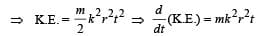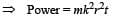Test: Single Correct MCQs: Work, Energy and Power | JEE Advanced - Question 4

A spring of force-constant k is cut into two pieces such that one piece is double the length of the other. Then the long piece will have a force-constant of

Detailed Solution for Test: Single Correct MCQs: Work, Energy and Power | JEE Advanced - Question 4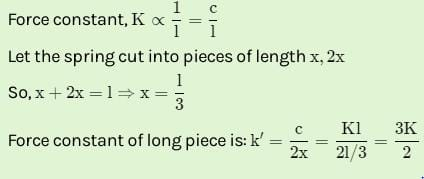Test: Single Correct MCQs: Work, Energy and Power | JEE Advanced - Question 5

A wind-powered generator converts wind energy into electrical energy. Assume that the generator converts a fixed fraction of the wind energy intercepted by its blades into electrical energy. For wind speed v, the electrical power output will be proportional to

Detailed Solution for Test: Single Correct MCQs: Work, Energy and Power | JEE Advanced - Question 5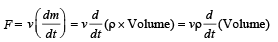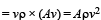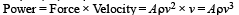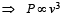Test: Single Correct MCQs: Work, Energy and Power | JEE Advanced - Question 6

A particle, which is constrained to move along the x-axis, is subjected to a force in the same direction which varies with the distance x of the particle from the origin as F(x) = –kx + ax3.  Here k and a are positive constants. For x≥ 0 , the functional form of the potential energy U(x) of the particle is

Detailed Solution for Test: Single Correct MCQs: Work, Energy and Power | JEE Advanced - Question 6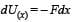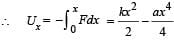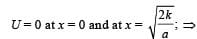we have potential energy zero twice (out of which one is at origin).
Also, when we put x = 0 in the given function,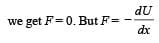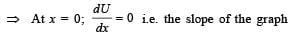should be zero. These characteristics are represented by (d).

Test: Single Correct MCQs: Work, Energy and Power | JEE Advanced - Question 7

An ideal spring with spring-constant k is hung from the ceiling and a block of mass M is attached to its lower end.
The mass is released with the spring initially unstretched.
Then the maximum extension in the spring is

Detailed Solution for Test: Single Correct MCQs: Work, Energy and Power | JEE Advanced - Question 7

The above situation can also be looked upon as the decrease in the gravitational potential energy of spring mass system is equal to the gain in spring elastic potential energy.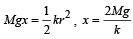Test: Single Correct MCQs: Work, Energy and Power | JEE Advanced - Question 8

If  W1, W2 and W3 represent the work done in moving a particle from A to B along three different paths 1,2 and 3 respectively (as shown) in the gravitational field of a point mass m, find the correct relation between W1, W2 and W3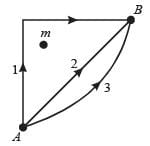Detailed Solution for Test: Single Correct MCQs: Work, Energy and Power | JEE Advanced - Question 8

Note : In a conservative field work done does not depend on the path. The gravitational field is a conservative field.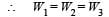Test: Single Correct MCQs: Work, Energy and Power | JEE Advanced - Question 9

A particle is acted by a force F = kx, where k is a +ve constant. Its potential energy at x = 0 is zero. Which curve correctly represents the variation of potential energy of the block with respect t o x

Detailed Solution for Test: Single Correct MCQs: Work, Energy and Power | JEE Advanced - Question 9

We know that ΔU = – W for conservative forces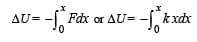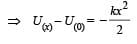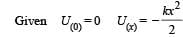Test: Single Correct MCQs: Work, Energy and Power | JEE Advanced - Question 10

A block (B) is attached to two unstretched springs S1 and S2 with spring constants k and 4k, respectively (see fig. I).
The other ends are attached to identical supports M1 and M2 not attached to the walls. The springs and supports have negligible mass. There is no friction anywhere. The block B is displaced towards wall 1 by a small distance x (figure II) and released. The block returns and moves a maximum distance y towards wall 2. Displacements x and y are measured with respect to the equilibrium position of the block B. The ratio y/x is –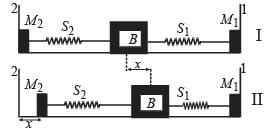Detailed Solution for Test: Single Correct MCQs: Work, Energy and Power | JEE Advanced - Question 10

When the block B is displaced towards wall 1, only spring S1 is compressed and S2 is in its natural state.
This happens because the other end of S2 is not attached to the wall but is free. Therefore the energy stored in the system = 1/2
k1 x2 . When the block is released, it will come back to the equilibrium position, gain momentum, overshoot to equilibrium position and move towards wall 2. As this happens, the spring S1 comes to its natural length and S2 gets compressed. As there are no frictional forces involved, the P.E. stored in the spring S1 gets stored as the P.E. of spring S2 when the block B reaches its extreme position after compressing S2 by y.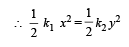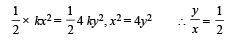Test: Single Correct MCQs: Work, Energy and Power | JEE Advanced - Question 11

Two small particles of equal masses start moving in opposite directions from a point A in a horizontal circular orbit. Their tangential velocities are v  and 2v, respectively, as shown in the figure. Between collisions, the particles move with constant speeds. After making how many elastic collisions, other than that at A, these two particles will again reach the point A?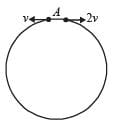Detailed Solution for Test: Single Correct MCQs: Work, Energy and Power | JEE Advanced - Question 11

Let the radius of the circle be r. Then the two distance travelled by the two particles before first collision is 2πr. Therefore

2v ´ t + v ´ t =2pr

where t is the time taken for first collision to occur.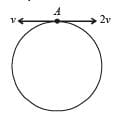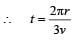Distance travelled by particle with velocity v is equal to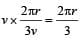Therefore the collision occurs at B.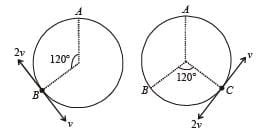As the collision is elastic and the particles have equal masses, the velocities will interchange as shown in the figure. According to the same reasoning as above, the 2nd collision will take place at C and the velocities will again interchange.
With the same reasoning the 3rd collision will occur at the point A. Thus there will be two elastic collisions before the particles again reach at A.

Test: Single Correct MCQs: Work, Energy and Power | JEE Advanced - Question 12

A piece of wire is bent in the shape of a parabola y = kx2 (y-axis vertical) with a bead of mass m on it. The bead can slide on the wire without friction. It stays at the lowest point of the parabola when the wire is at rest. The wire is now accelerated parallel to the x-axis with a constant acceleration a. The distance of the new equilibrium position of the bead, where the bead can stay at rest with respect to the wire, from the y-axis is

Detailed Solution for Test: Single Correct MCQs: Work, Energy and Power | JEE Advanced - Question 12

The forces acting on the bead as seen by the observer in the accelerated frame are : (a) N ; (b) mg ; (c) ma (pseudo force).
Let θ is the angle which the tangent at P makes with the X- axis. As the bead is in equilibrium with respect to the wire, therefore N sinθ = ma and N cos θ = mg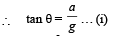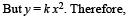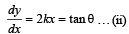From (i) & (ii)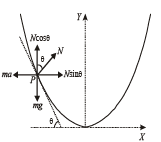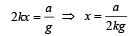Test: Single Correct MCQs: Work, Energy and Power | JEE Advanced - Question 13

A block of mass 2 kg is free to move along the x-axis. It is at rest and from t = 0 onwards it is subjected to a time-dependent force F(t) in the x direction. The force F(t) varies with t as shown in the figure. The kinetic energy of the block after 4.5 seconds is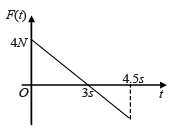Detailed Solution for Test: Single Correct MCQs: Work, Energy and Power | JEE Advanced - Question 13

Area under F – t graph gives the impulse or the change in the linear momentum of the body. As the initial velocity (and therefore the initial linear momentum) of the body is zero, the area under F – t graph gives the final linear momentum of the body.
Area of ΔAOB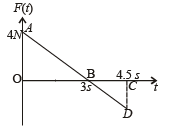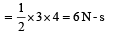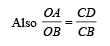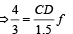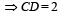Test: Single Correct MCQs: Work, Energy and Power | JEE Advanced - Question 14

The work done on a particle of mass m by a force,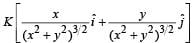(K being a constant of appropriate dimensions), when the particle is taken from the point (a, 0) to the point (0, a) along a circular path of radius a about the origin in the x – y plane is

Detailed Solution for Test: Single Correct MCQs: Work, Energy and Power | JEE Advanced - Question 14

Let us consider a point on the circle The equation of circle is x2 + y2 = a2 The force is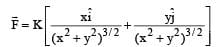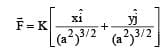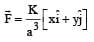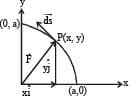The force acts radially outwards as shown in the figure and the displacement is tangential to the circular path.
Therefore the angle between the force and displacement is 90° and W = 0 option (d) is correct.

Test: Single Correct MCQs: Work, Energy and Power | JEE Advanced - Question 15

A tennis ball is dropped on a horizontal smooth surface. It bounces back to its original position after hitting the surface.
The force on the ball during the collision is proportional to the length of compression of the ball. Which one of the following sketches describes the variation of its kinetic energy K with time t most appropriately? The figure are only illustrative and not to the scale.

Detailed Solution for Test: Single Correct MCQs: Work, Energy and Power | JEE Advanced - Question 15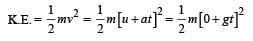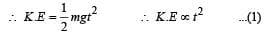First the kinetic energy will increase as per eq (1). As the balls touches the ground it starts deforming and loses its K.E. (K.E. converting into elastic potential energy). When the deformation is maximum, K.E. = 0.
The ball then again regain its shape when its elastic potential energy changes into K.E. As the ball moves up it loses K.E. and gain gravitational potential energy.
These characteristics are according to graph (b).

*Multiple options can be correct
Test: Single Correct MCQs: Work, Energy and Power | JEE Advanced - Question 16

A body is moved along a straight line by a machine delivering constant power. The distance moved by the body in time t is proportional to

Detailed Solution for Test: Single Correct MCQs: Work, Energy and Power | JEE Advanced - Question 16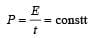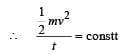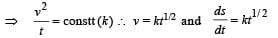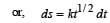By integrating, we get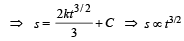*Multiple options can be correct
Test: Single Correct MCQs: Work, Energy and Power | JEE Advanced - Question 17

A uniform chain of length L and mass M is lying on a smooth table and one third of its length is hanging vertically down over the edge of the table. If g is acceleration due to gravity, the work required to pull the hanging part on to the table is

Detailed Solution for Test: Single Correct MCQs: Work, Energy and Power | JEE Advanced - Question 17

The hanging part of the chain which is to be pulled up can be considered as a point mass situated at the centre of the hanging part. The equivalent diagram is drawn.
Note : The work done in bringing the mass up will be equal to the change in potential energy of the mass.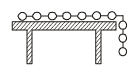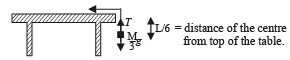W = Change in potential energy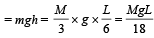*Multiple options can be correct
Test: Single Correct MCQs: Work, Energy and Power | JEE Advanced - Question 18

A particle is acted upon by a force of constant magnitude which is always perpendicular to the velocity of the particle.
The motion of the particle takes place in a plane. It follows that :

Detailed Solution for Test: Single Correct MCQs: Work, Energy and Power | JEE Advanced - Question 18

When the force is perpendicular to the velocity and constant in magnitude then the force acts as a centripetal force, and the body moves in a circular path. The force is constant in magnitude, this show the speed is not changing and hence kinetic energy will remain constant.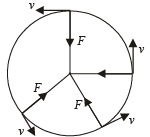Note : The velocity changes continuously due to change in the direction. The acceleration also changes continuously due to change in direction.

*Multiple options can be correct
Test: Single Correct MCQs: Work, Energy and Power | JEE Advanced - Question 19

A force F = - K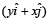(where K is a positive constant) acts on a particle moving in the xy plane. Starting from the origin, the particle is taken along the positive x axis to the point (a, 0), and then parallel to the y axis to the point (a, a), The total work done by the force F on the particle is

Detailed Solution for Test: Single Correct MCQs: Work, Energy and Power | JEE Advanced - Question 19

The expression of work done by the variable force F on the particle is given by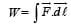In going from (0, 0) to (a, 0), the coordinate of x varies from 0 to 'a', while that of y remains zero. Hence, the work done along this path is :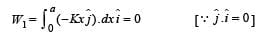In going from (a, 0) to (a, a) the coordinate of x remains constant (= a) while that of y changes from 0 to 'a'.
Hence, the work done along this path is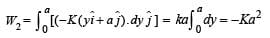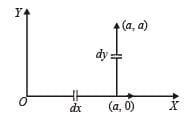Hence, W = W1 + W2 = – ka2

*Multiple options can be correct
Test: Single Correct MCQs: Work, Energy and Power | JEE Advanced - Question 20

A stone tied to a string of length L is whirled in a vertical circle with the other end of the string at the centre. At a certain instant of time, the stone is at its lowest position, and has a speed u. The magnitude of  the change in its velocity as it reaches a position where the string is horizontal is

Detailed Solution for Test: Single Correct MCQs: Work, Energy and Power | JEE Advanced - Question 20

Applying the principle of conservation of energy (K.E.)B + (P.E.)B = (K.E.)A + (P.E.)A,

we get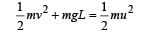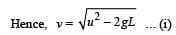Change in velocity =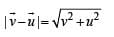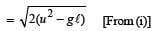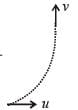*Multiple options can be correct
Test: Single Correct MCQs: Work, Energy and Power | JEE Advanced - Question 21

A small ball starts moving from A over a fixed track as shown in the figure. Surface AB has friction. From A to B the ball rolls without slipping. Surface BC is frictionless. KA, KB and KC are kinetic energies of the ball at A, B and C, respectively.
Then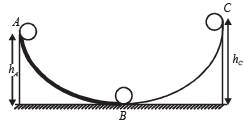Detailed Solution for Test: Single Correct MCQs: Work, Energy and Power | JEE Advanced - Question 21

At point A, potential energy of the ball = mghA

At point B, potential energy of the ball = 0

At point C, potential energy of the ball = mghC

Total energy at point A, EA = KA + mghA

Total energy at point B, EB = KB

Total energy at point C, EC = KC + mghC

According to the law of conservation of energy.

EA = EB = EC ... (i)

EA = EB ⇒ EC  > KC ...(ii)

EA = EC

KA + mghA = KB + mghC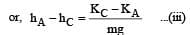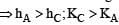Option (b) is correct

From (i),(ii) and (iv), we get hA> hC; KB > KC.Option (a) is correct.

## Physics For JEE

258 videos|633 docs|256 tests
 Use Code STAYHOME200 and get INR 200 additional OFF Use Coupon Code
Information about Test: Single Correct MCQs: Work, Energy and Power | JEE Advanced Page
In this test you can find the Exam questions for Test: Single Correct MCQs: Work, Energy and Power | JEE Advanced solved & explained in the simplest way possible. Besides giving Questions and answers for Test: Single Correct MCQs: Work, Energy and Power | JEE Advanced, EduRev gives you an ample number of Online tests for practice

## Physics For JEE

258 videos|633 docs|256 tests